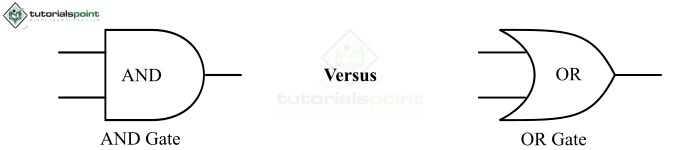# Difference Between AND Gate and OR Gate

In digital electronics, the logic gates are the basic building blocks of all digital circuits that act as the switching devices and determine whether the input signal may pass through to the output of the digital circuits.

As the name implies, a logic gate is a digital circuit used to perform several logical operations in a digital device or circuit. A logic gate can accept one or multiple inputs but produces only a single output. Where, the output of a logic gate is determined by the combination of input signals. The operation of the logic gates is based on the Boolean algebra.

Logic gates are found in every digital and electronic device such as smartphone, laptops, computers, memories, video games, etc.

There are many types logic gates available such as AND gate, OR gate, NOT gate, NAND gate, NOR gate, XOR gate, XNOR gate, etc.In this article, we will highlight the significant differences between AND gate and OR gate by considering different parameters. Let's start with some basics of both AND gate and OR gate so that it becomes easier to understand the differences between them.

## What is an AND Gate?

An AND gate the type of logic gate which performs the logical multiplication of binary input signals to produce an output. The operation performed by the AND gate is referred as the AND operation, which is similar to an ordinary multiplication of 1s and 0s. The AND gate is a type of basic logic gate.

In other words, we may define the AND gate as a digital logic gate which produces an output ‘1’ when it’s all the inputs are high, i.e. ‘1’, and the output of the AND gate is low, i.e. ‘0’in all other cases. The AND operation is usually denoted by a dot (.).

The logical expression of a two input AND gate is given by

$$Y\:=\:A\cdot\:B$$

Which is read as Y equals to A AND B.

The truth table of a two input AND gate is given below.

Inputs Output
A B Y = A.B
0 0 0
0 1 0
1 0 0
1 1 1

The AND gate is used in several digital devices and systems like in data transmission, digital measuring devices, alarm circuits, automatic temperature-control circuits, push button logic implementation, etc.

## What is an OR Gate?

An OR gate is a type of logic gate which performs the logical addition of binary input signals to produce an output signal. An OR gate may have single or multiple inputs but it has only a single output. A plus symbol (+) is used to represent the OR operation.

In other words, we may also define the OR gate as a logic gate which produces a high output, i.e. ‘1’, if it’s any one of the inputs is high, i.e. ‘1’, and the output is low (0), only if all the inputs of the OR gate are low (0).

The logical expression of a two input OR gate is given by,

$$Y\:=\:A\:+\:B$$

Which is read as Y equals to A OR B.

The truth table of a two input OR gate is given below.

Inputs Output
A B Y = A+B
0 0 0
0 1 1
1 0 1
1 1 1

The OR gates are used in different automatic digital circuits such as in door-bell switches, temperature detection circuits, pressure detection circuits, etc.

## Difference Between AND Gate and OR Gate

Both AND gate and OR Gate are basic logic gates. There are several differences between AND gate and OR gate that are highlighted in the following table −

Basis of Difference AND Gate OR Gate
Definition A logic gate which performs the logical multiplication of binary input signals and produces a single binary output signal is known as AND gate. A logic gate which performs the logical additions of binary input signals to produce a single binary output signal is known as OR gate.
Representation The operation of an AND gate is represented by a dot (.). The operation of an OR gate is represented by a plus (+).
Logical Expression

The logical expression of a two input AND is given by,

$$Y\:=\:A\:\cdot\:B$$

The logical expression of a multiinput AND gate is,

$$Y\:=\:A\cdot\:B\cdot\:C\dotso$$

The logical expression of a two input OR gate is given by,

$$Y\:=\:A\:+\:B$$

The logical expression of a multiinput OR gate is,

$$Y\:=\:A\:+\:B+\:C\:+\dotso$$
Low Output AND gate produces a low (0) output, if any one of its inputs becomes low (0). OR gate produces a low (0) output only if all its inputs are low (0).
High Output AND gate produces a high (1) output only if all its inputs are high (1). If one or more inputs of an OR gate is high, then the OR gate produces a high output (1).
Electric circuit analogy A two input AND gate is analogous to the two electric switches connected in series. A two input OR gate is analogous to the two electric switches connected in parallel.
Applications The practical applications of AND gate are in data transmission, push button implementation, digital alarm circuits, etc. OR gates are used in automatic digital control circuits such as in temperature control circuits, pressure control circuits, etc.

## Conclusion

The most significant difference between an AND gate and an OR gate is that an AND gate gives output high, if all its inputs are high, whereas an OR gate gives output high if any one of its inputs is high. Both types of gates play a crucial role in many digital electronic circuits and devices.

Updated on: 12-Apr-2023

4K+ Views